2019-01-15 18:40:41 qq_42887760 阅读数 125
• ###### MATLAB图像处理

MATLAB图像处理课程

4588 人正在学习 去看看 李立宗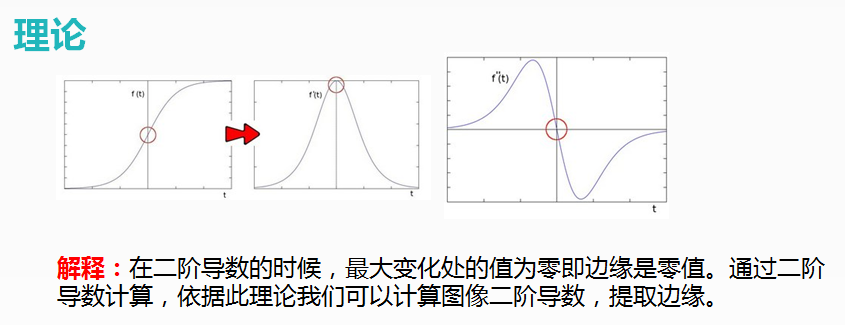# Laplance算子

• 二阶导数我不会，别担心 ->拉普拉斯算子(Laplance operator)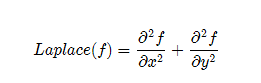• Opencv已经提供了相关API - cv::Laplance

# API使用cv::Laplacian

```Laplacian( InputArray src, OutputArray dst, int depth, //深度CV_16S int kisze, // 3 double scale = 1, double delta =0.0, int borderType = 4 )```

# 处理流程

• 高斯模糊 – 去噪声GaussianBlur()
• 转换为灰度图像cvtColor()
• 拉普拉斯 – 二阶导数计算Laplacian()
• 取绝对值convertScaleAbs()
• 显示结果

# 代码示例

``````#include <opencv2/opencv.hpp>
#include <iostream>
using namespace cv;

int main( int, char** argv )
{
Mat src, gblur_src,gray_src,laplace_src,dst;
// 1.加载源图像src
if( src.empty()){ //检测图片
return -1;
}
imshow("input",src);//输出图像

// 2. 先模糊，为了去噪声。将cv :: GaussianBlur应用于我们的图像以减少噪音（内核大小= 3）
GaussianBlur( src, gblur_src, Size(3,3), 0, 0, BORDER_DEFAULT );
// 3. 将滤波后的图像转换为灰度图像：
cvtColor( gblur_src, gray_src, COLOR_RGB2GRAY );

// 4.分别用 Laplance 算子进行图像边缘化处理
Laplacian(gray_src, laplace_src, CV_16S, 3);//参数意思与上述的 Sobel Scharr 函数一致
convertScaleAbs(laplace_src, laplace_src);
imshow("Laplance Demo", laplace_src);

threshold(laplace_src, dst, 0, 255, THRESH_OTSU | THRESH_BINARY);//二值化，边缘显示的更清晰
imshow("dst", dst);

waitKey(0);
return 0;
}
``````

# 运行截图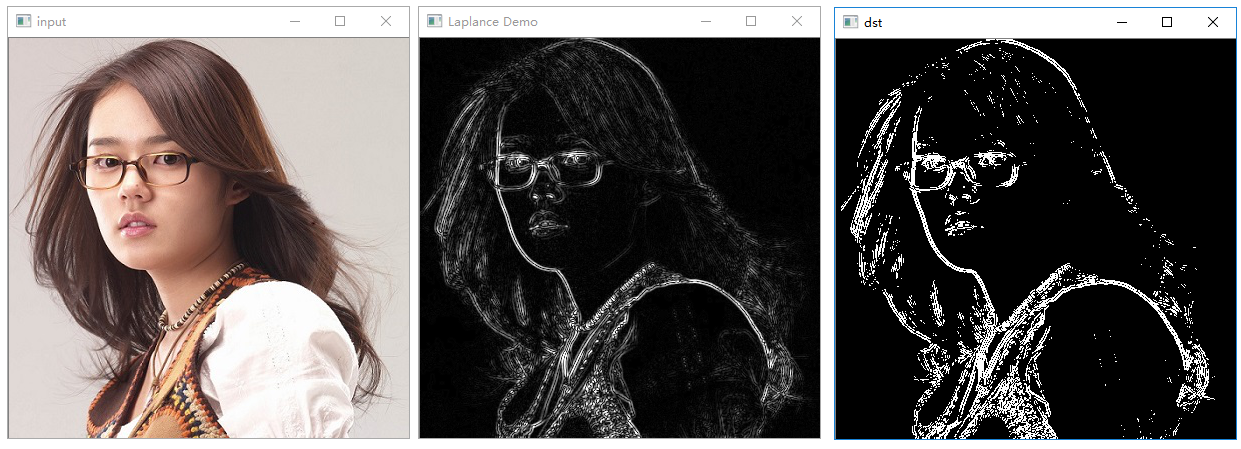### 参考博客

2018-08-06 22:17:16 Eastmount 阅读数 7265
• ###### MATLAB图像处理

MATLAB图像处理课程

4588 人正在学习 去看看 李立宗

1.灰度转换：将彩色图片转换为灰度图像，常见的R=G=B=像素平均值。
2.高斯平滑和中值滤波：去除噪声。
3.Sobel算子：提取图像边缘轮廓，X方向和Y方向平方和开跟。
4.二值化处理：图像转换为黑白两色，通常像素大于127设置为255，小于设置为0。
5.膨胀和细化：放大图像轮廓，转换为一个个区域，这些区域内包含车牌。
6.通过算法选择合适的车牌位置，通常将较小的区域过滤掉或寻找蓝色底的区域。
7.标注车牌位置，如果是花儿、人脸、牛角，可能需要特征提取和训练。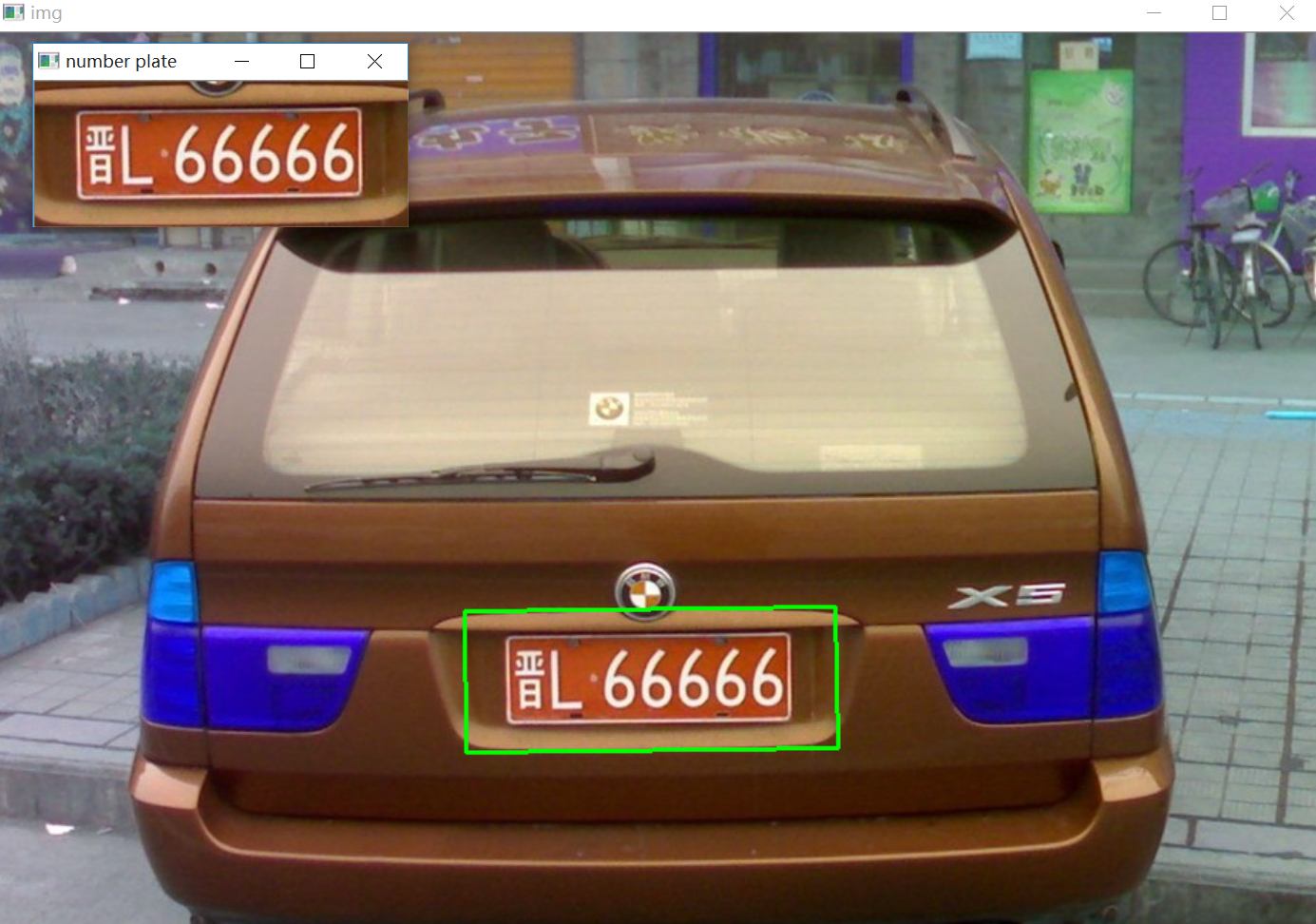https://blog.csdn.net/column/details/eastmount-mfc.html

PSS：2019年1~2月作者参加了CSDN2018年博客评选，希望您能投出宝贵的一票。我是59号，Eastmount，杨秀璋。投票地址：https://bss.csdn.net/m/topic/blog_star2018/index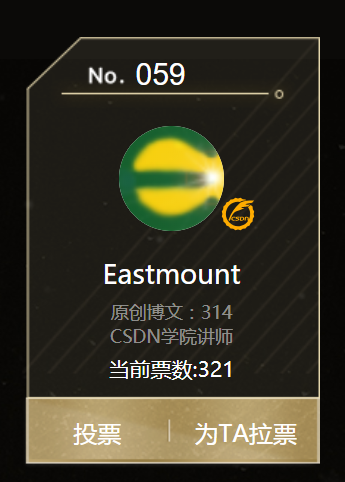## 一、读取图像及灰度转换

``````#encoding:utf-8
import cv2
import numpy as np
import matplotlib.pyplot as plt

#读取图片
imagePath = '10.jpg'

#而matplotlib的imshow默认则是以RGB格式展示
#所以此处我们必须对图片的通道进行转换
lenna_img = cv2.cvtColor(img,cv2.COLOR_BGR2RGB)

#灰度图像处理
GrayImage = cv2.cvtColor(img,cv2.COLOR_BGR2GRAY)
print(u"读入lenna图的shape为", GrayImage.shape)

#显示图形
titles = ['Source Image','Gray Image']
images = [lenna_img, GrayImage]
for i in xrange(2):
plt.subplot(1,2,i+1),plt.imshow(images[i],'gray')
plt.title(titles[i])
plt.xticks([]),plt.yticks([])
plt.show()

cv2.imshow('Gray.bmp', GrayImage)
cv2.waitKey(0)

``````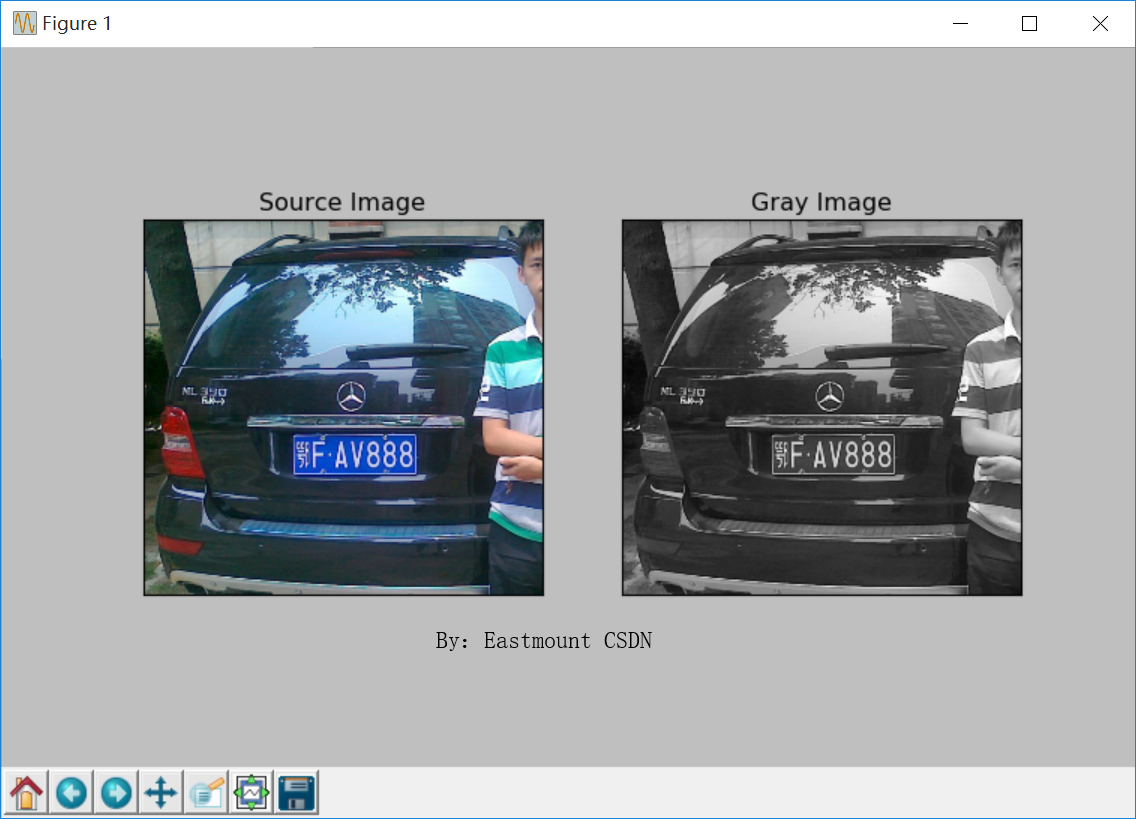## 二、高斯平滑和中值滤波去噪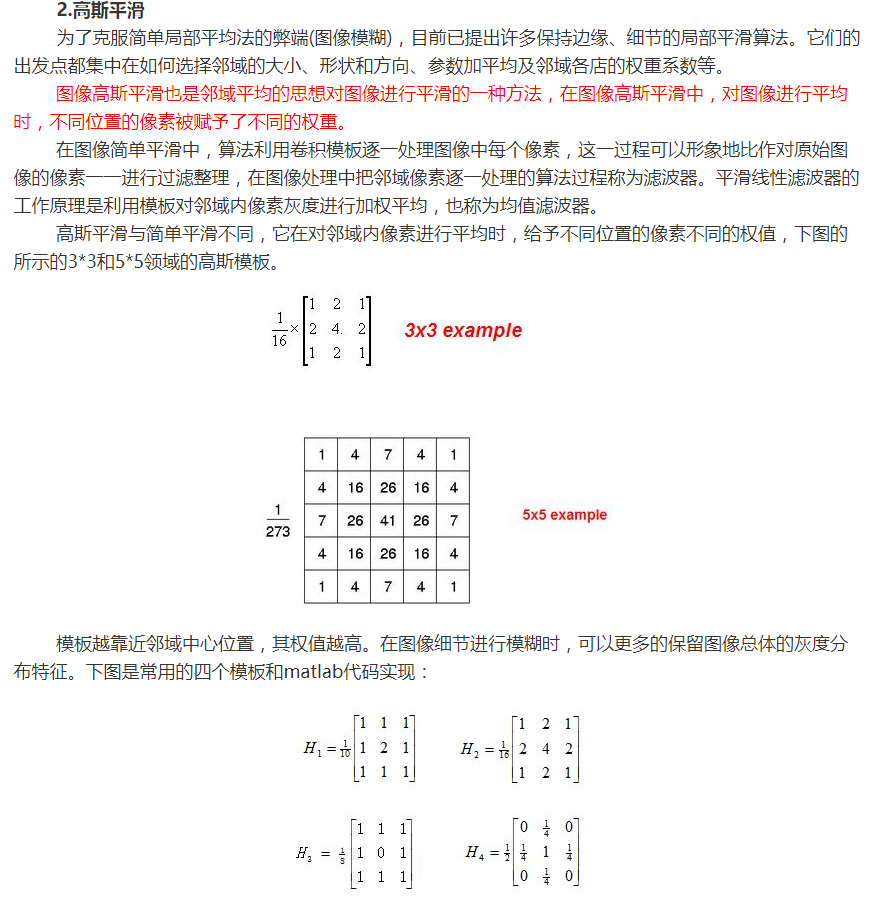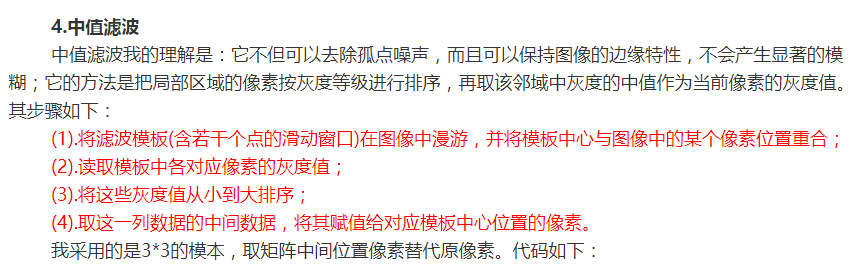``````#encoding:utf-8
import cv2
import numpy as np
import matplotlib.pyplot as plt

#读取图片
imagePath = '10.jpg'

#而matplotlib的imshow默认则是以RGB格式展示
#所以此处我们必须对图片的通道进行转换
lenna_img = cv2.cvtColor(img,cv2.COLOR_BGR2RGB)

#灰度图像处理
GrayImage = cv2.cvtColor(img,cv2.COLOR_BGR2GRAY)
print(u"读入lenna图的shape为", GrayImage.shape)

#直方图均衡化
#equ = cv2.equalizeHist(gray)

#高斯平滑
Gaussian = cv2.GaussianBlur(GrayImage, (3, 3), 0, 0, cv2.BORDER_DEFAULT)
#Gaussian = cv2.GaussianBlur(GrayImage, (9, 9),0)

#中值滤波
Median = cv2.medianBlur(Gaussian, 5)

#显示图形
titles = ['Source Image','Gray Image', 'Gaussian Image', 'Median Image']
images = [lenna_img, GrayImage, Gaussian, Median]
for i in xrange(4):
plt.subplot(2,2,i+1),plt.imshow(images[i],'gray')
plt.title(titles[i])
plt.xticks([]),plt.yticks([])
plt.show()  ``````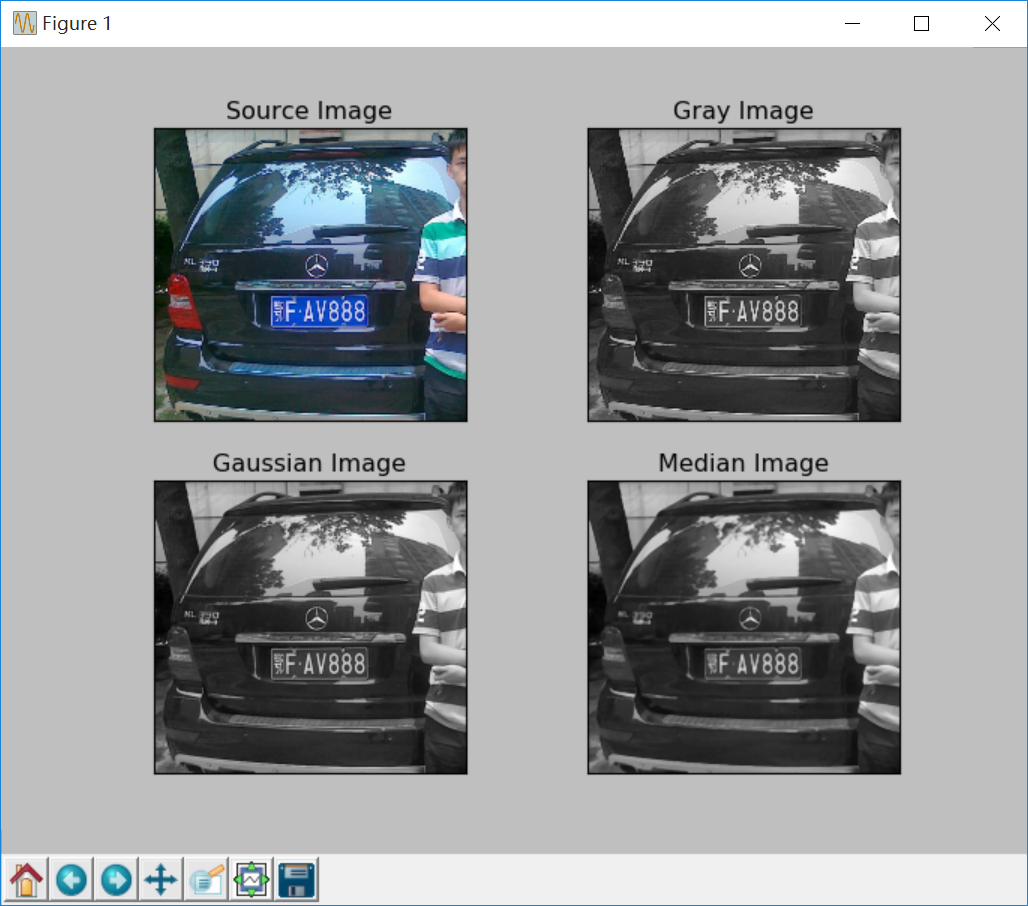## 三、Sobel算子提取轮廓和二值化处理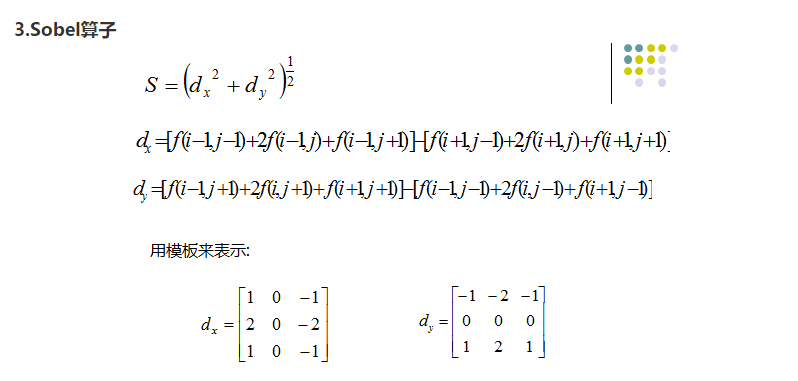``````#encoding:utf-8
import cv2
import numpy as np
import matplotlib.pyplot as plt

#读取图片
imagePath = '10.jpg'

lenna_img = cv2.cvtColor(img,cv2.COLOR_BGR2RGB)

#灰度图像处理
GrayImage = cv2.cvtColor(img,cv2.COLOR_BGR2GRAY)
print(u"读入lenna图的shape为", GrayImage.shape)

#直方图均衡化
#equ = cv2.equalizeHist(gray)

# 高斯平滑
Gaussian = cv2.GaussianBlur(GrayImage, (3, 3), 0, 0, cv2.BORDER_DEFAULT)

# 中值滤波
Median = cv2.medianBlur(Gaussian, 5)

# Sobel算子 XY方向求梯度
x = cv2.Sobel(Median, cv2.CV_8U, 1, 0, ksize = 3) #X方向
y = cv2.Sobel(Median, cv2.CV_8U, 0, 1, ksize = 3) #Y方向
absX = cv2.convertScaleAbs(x)   # 转回uint8
absY = cv2.convertScaleAbs(y)
Sobel = cv2.addWeighted(absX, 0.5, absY, 0.5,0)
cv2.imshow('dilation2', Sobel)
cv2.waitKey(0)

# 二值化处理 周围像素影响
ret, Binary = cv2.threshold(Sobel, 170, 255, cv2.THRESH_BINARY)
cv2.imshow('dilation2',Binary)
cv2.waitKey(0)

#显示图形
titles = ['Source Image','Gray Image', 'Gaussian Image', 'Median Image',
'Sobel Image', 'Binary Image']
images = [lenna_img, GrayImage, Gaussian, Median, Sobel, Binary]
for i in xrange(6):
plt.subplot(2,3,i+1),plt.imshow(images[i],'gray')
plt.title(titles[i])
plt.xticks([]),plt.yticks([])
plt.show()

``````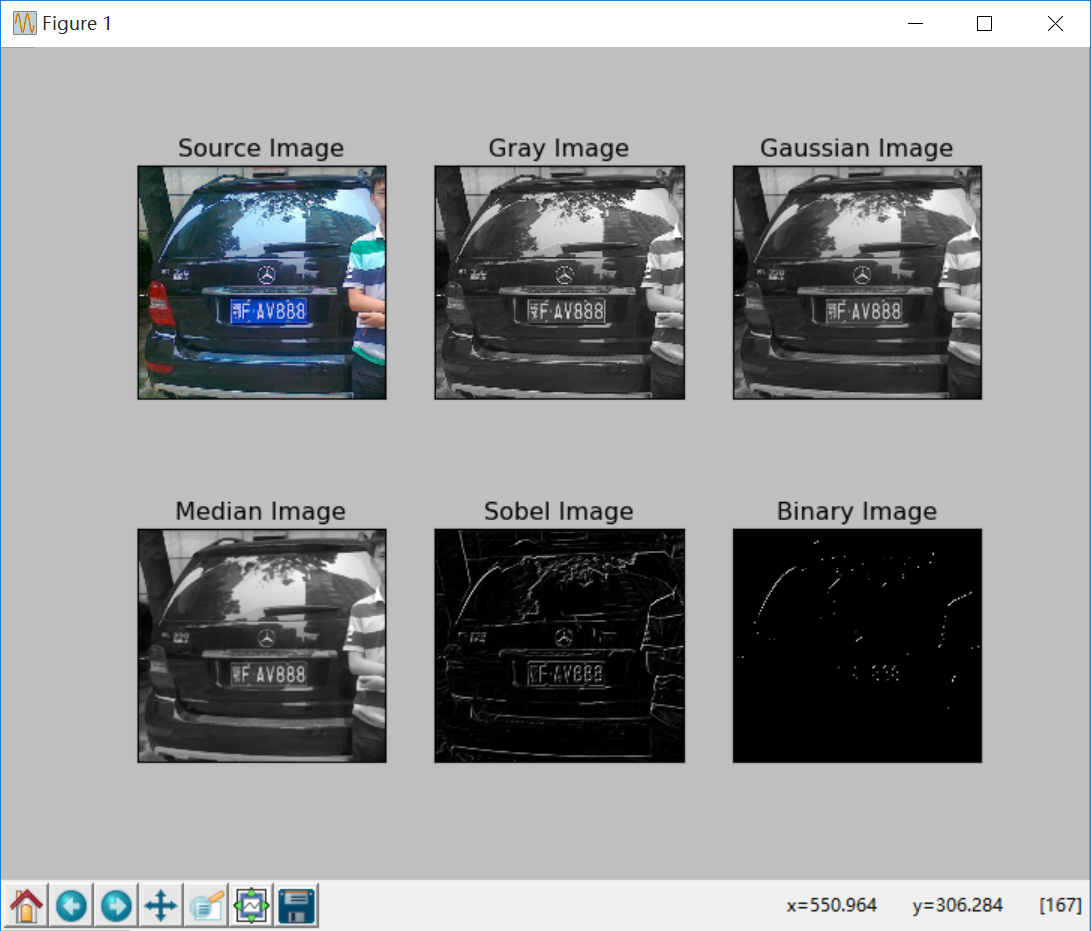## 四、膨胀和腐蚀处理

``````#encoding:utf-8
import cv2
import numpy as np
import matplotlib.pyplot as plt

#读取图片
imagePath = '10.jpg'

lenna_img = cv2.cvtColor(img,cv2.COLOR_BGR2RGB)

#灰度图像处理
GrayImage = cv2.cvtColor(img,cv2.COLOR_BGR2GRAY)
print(u"读入lenna图的shape为", GrayImage.shape)

#直方图均衡化
#equ = cv2.equalizeHist(gray)

#高斯平滑 去噪
Gaussian = cv2.GaussianBlur(GrayImage, (3, 3), 0, 0, cv2.BORDER_DEFAULT)
#Gaussian = cv2.GaussianBlur(GrayImage, (9, 9),0)

#中值滤波
Median = cv2.medianBlur(Gaussian, 5)

#Sobel算子 XY方向求梯度 cv2.CV_8U
x = cv2.Sobel(Median, cv2.CV_32F, 1, 0, ksize = 3) #X方向
y = cv2.Sobel(Median, cv2.CV_32F, 0, 1, ksize = 3) #Y方向
#absX = cv2.convertScaleAbs(x)   # 转回uint8
#absY = cv2.convertScaleAbs(y)
#Sobel = cv2.addWeighted(absX, 0.5, absY, 0.5, 0)
cv2.imshow('dilation2', Sobel)
cv2.waitKey(0)

#二值化处理 周围像素影响
blurred = cv2.GaussianBlur(Sobel, (9, 9),0) #再进行高斯去噪
#注意170可以替换的
ret, Binary = cv2.threshold(blurred , 90, 255, cv2.THRESH_BINARY)
cv2.imshow('dilation2', Binary)
cv2.waitKey(0)

#膨胀和腐蚀操作的核函数
element1 = cv2.getStructuringElement(cv2.MORPH_RECT, (9, 1))
element2 = cv2.getStructuringElement(cv2.MORPH_RECT, (9, 7))

# 膨胀让轮廓突出
Dilation = cv2.dilate(Binary, element2, iterations = 1)
# 腐蚀去掉细节
Erosion = cv2.erode(Dilation, element1, iterations = 1)
# 再次膨胀
Dilation2 = cv2.dilate(Erosion, element2,iterations = 3)
cv2.imshow('Dilation2 ', Dilation2)
cv2.waitKey(0)

#显示图形
titles = ['Source Image','Gray Image', 'Gaussian Image', 'Median Image',
'Sobel Image', 'Binary Image', 'Dilation Image', 'Erosion Image', 'Dilation2 Image']
images = [lenna_img, GrayImage, Gaussian,
Median, Sobel, Binary,
Dilation, Erosion, Dilation2]
for i in xrange(9):
plt.subplot(3,3,i+1),plt.imshow(images[i],'gray')
plt.title(titles[i])
plt.xticks([]),plt.yticks([])
plt.show()

``````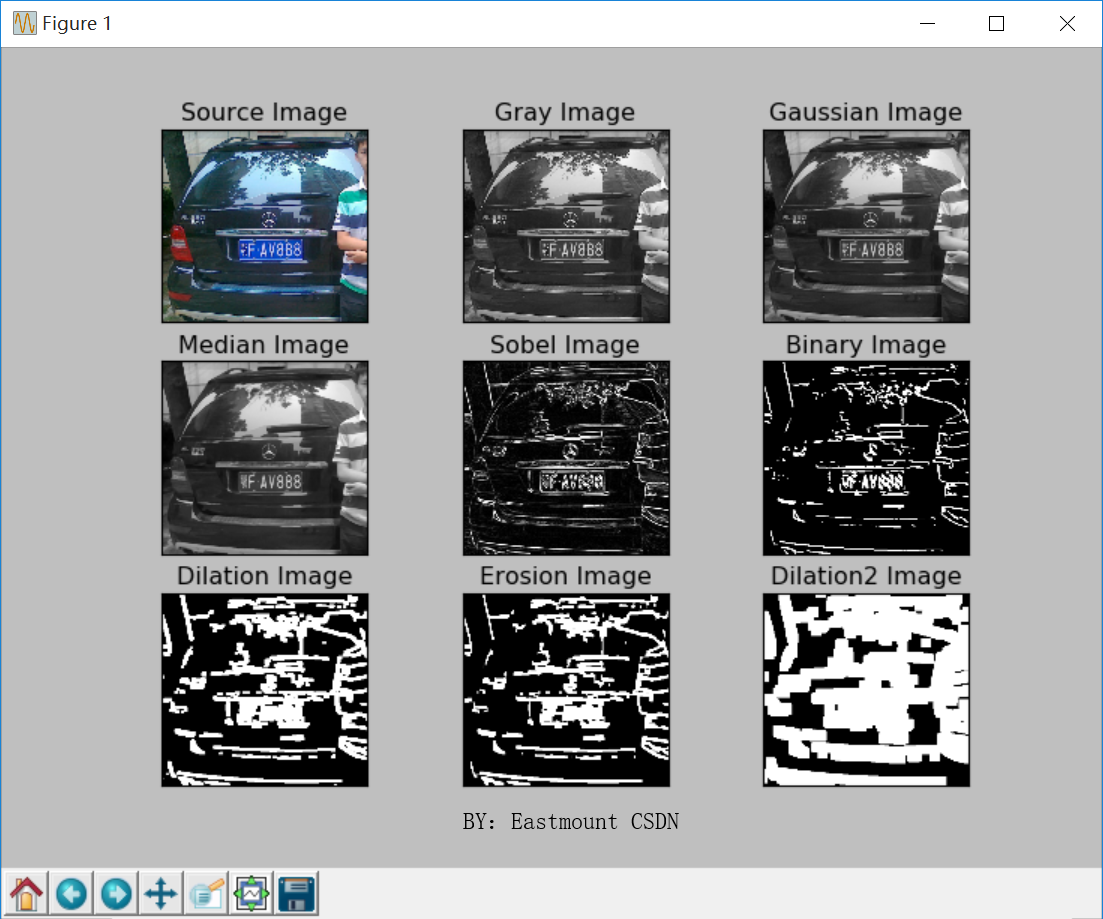## 五、指定算法选择车牌区域

``````#encoding:utf-8
#BY：Eastmount CSDN 2018-08-06
import cv2
import numpy as np
import matplotlib.pyplot as plt

#读取图片
imagePath = '10.jpg'

lenna_img = cv2.cvtColor(img,cv2.COLOR_BGR2RGB)

#灰度图像处理
GrayImage = cv2.cvtColor(img,cv2.COLOR_BGR2GRAY)
print(u"读入lenna图的shape为", GrayImage.shape)

#直方图均衡化
#equ = cv2.equalizeHist(gray)

#高斯平滑 去噪
Gaussian = cv2.GaussianBlur(GrayImage, (3, 3), 0, 0, cv2.BORDER_DEFAULT)
#Gaussian = cv2.GaussianBlur(GrayImage, (9, 9),0)

#中值滤波
Median = cv2.medianBlur(Gaussian, 5)

#Sobel算子 XY方向求梯度 cv2.CV_8U
x = cv2.Sobel(Median, cv2.CV_32F, 1, 0, ksize = 3) #X方向
y = cv2.Sobel(Median, cv2.CV_32F, 0, 1, ksize = 3) #Y方向
#absX = cv2.convertScaleAbs(x)   # 转回uint8
#absY = cv2.convertScaleAbs(y)
#Sobel = cv2.addWeighted(absX, 0.5, absY, 0.5, 0)
cv2.imshow('dilation2', Sobel)
cv2.waitKey(0)

#二值化处理 周围像素影响
blurred = cv2.GaussianBlur(Sobel, (9, 9),0) #再进行一次高斯去噪
#注意170可以替换的
ret, Binary = cv2.threshold(blurred , 170, 255, cv2.THRESH_BINARY)
cv2.imshow('dilation2', Binary)
cv2.waitKey(0)

# 膨胀和腐蚀操作的核函数
element1 = cv2.getStructuringElement(cv2.MORPH_RECT, (9, 1))
element2 = cv2.getStructuringElement(cv2.MORPH_RECT, (9, 7))
# 膨胀一次，让轮廓突出
Dilation = cv2.dilate(Binary, element2, iterations = 1)
# 腐蚀一次，去掉细节
Erosion = cv2.erode(Dilation, element1, iterations = 1)
# 再次膨胀，让轮廓明显一些
Dilation2 = cv2.dilate(Erosion, element2,iterations = 3)
cv2.imshow('Dilation2 ', Dilation2)
cv2.waitKey(0)

##########################################

#建立一个椭圆核函数
kernel = cv2.getStructuringElement(cv2.MORPH_ELLIPSE, (25, 25))
#执行图像形态学, 细节直接查文档，很简单
closed = cv2.morphologyEx(Binary, cv2.MORPH_CLOSE, kernel)
closed = cv2.erode(closed, None, iterations=4)
closed = cv2.dilate(closed, None, iterations=4)
cv2.imshow('erode dilate', closed)
cv2.waitKey(0)

##########################################

#显示图形
titles = ['Source Image','Gray Image', 'Gaussian Image', 'Median Image',
'Sobel Image', 'Binary Image', 'Dilation Image', 'Erosion Image', 'Dilation2 Image']
images = [lenna_img, GrayImage, Gaussian,
Median, Sobel, Binary,
Dilation, Erosion, closed]
for i in xrange(9):
plt.subplot(3,3,i+1),plt.imshow(images[i],'gray')
plt.title(titles[i])
plt.xticks([]),plt.yticks([])
plt.show()

cv2.imshow('Gray', GrayImage)
cv2.waitKey(0)

"""

参数一：二值化图像
参数二：轮廓类型 检测的轮廓不建立等级关系
参数三：处理近似方法  例如一个矩形轮廓只需4个点来保存轮廓信息
"""
(_, cnts, _) = cv2.findContours(closed.copy(),
cv2.RETR_LIST,               #RETR_TREE
cv2.CHAIN_APPROX_SIMPLE)

#画出轮廓
c = sorted(cnts, key=cv2.contourArea, reverse=True)
print c

#compute the rotated bounding box of the largest contour
rect = cv2.minAreaRect(c)
print 'rectt', rect
Box = np.int0(cv2.boxPoints(rect))
print 'Box', Box

#draw a bounding box arounded the detected barcode and display the image
Final_img = cv2.drawContours(img.copy(), [Box], -1, (0, 0, 255), 3)

cv2.imshow('Final_img', Final_img)
cv2.waitKey(0)``````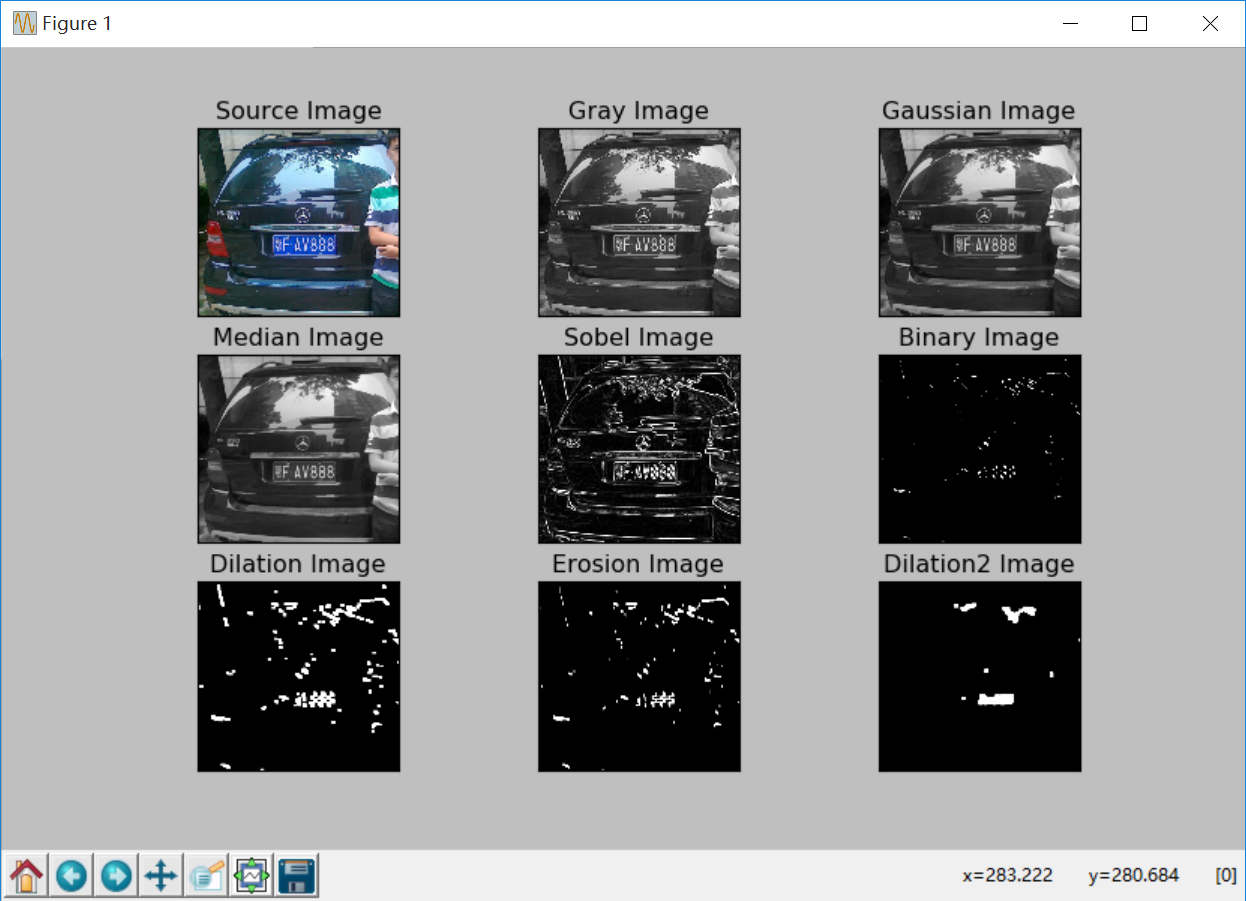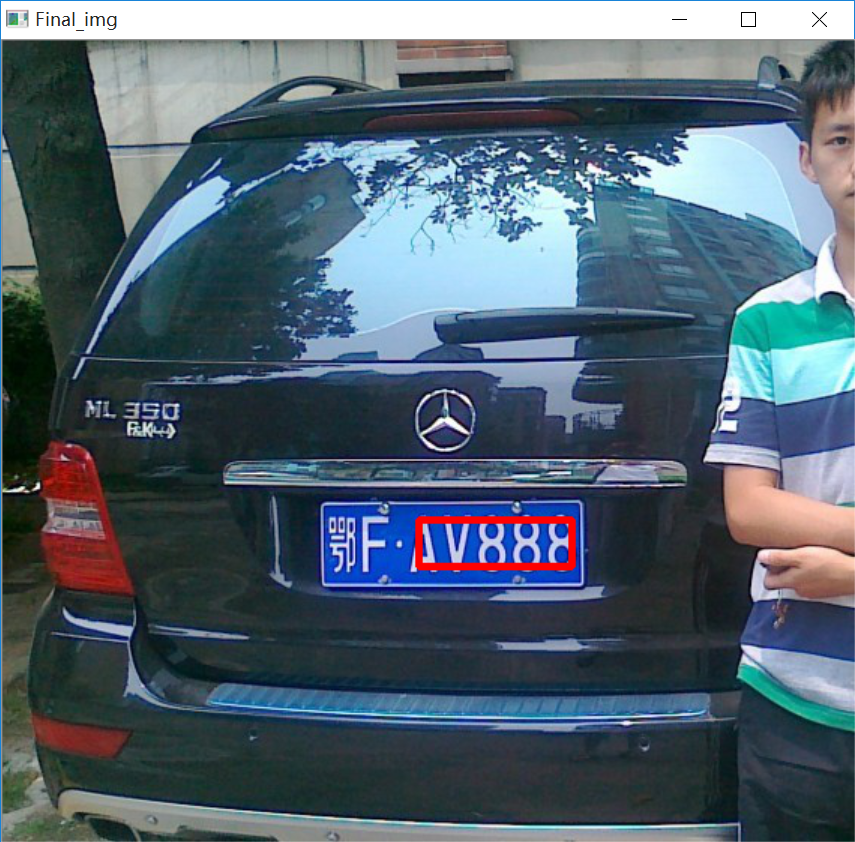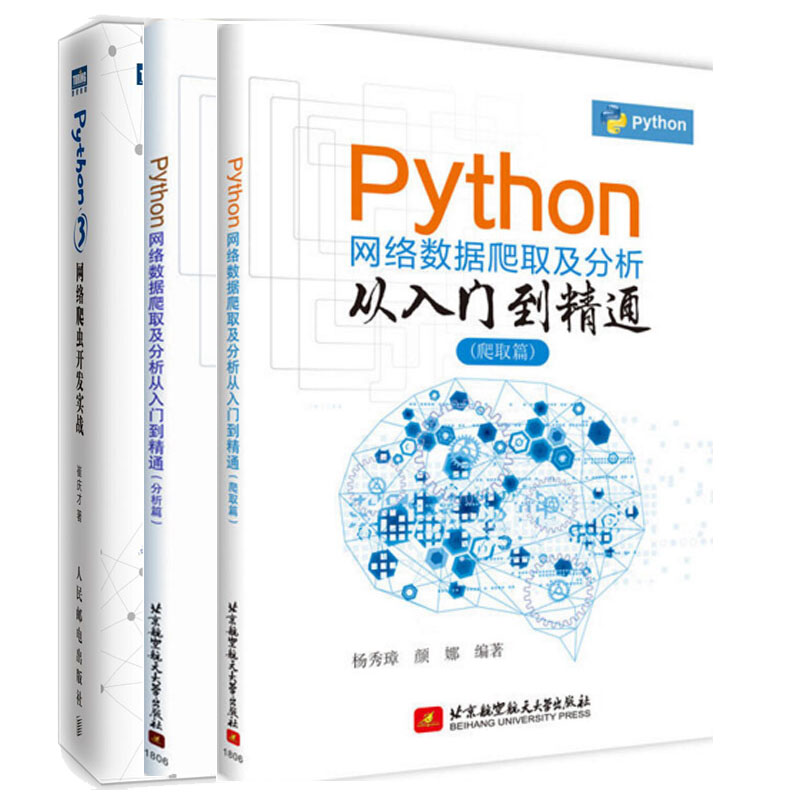## 本书主要包括上下两册：

《Python网络数据爬取及分析从入门到精通（爬取篇）》
《Python网络数据爬取及分析从入门到精通（分析篇）》

(By:Eastmount 2018-08-06 深夜11点  http://blog.csdn.net/eastmount/ )

2014-06-26 22:34:12 u013018721 阅读数 2057
• ###### MATLAB图像处理

MATLAB图像处理课程

4588 人正在学习 去看看 李立宗

①.基本原理

②.边缘检测算法有哪些

不难看出，边缘检测算法的核心是突出图像中变化率，无论目标与目标，还是目标与背景之间。这于导数的概念离奇的详尽。而对于离散的数字图像中，我们可以使用与求导

根据梯度模矩阵的不同求法，边缘检测算法可以分为两大类：一阶导数方式（梯度算子）和二阶导数方式

③.边缘检测在图像处理中的一般步骤其中第一步为滤波，因为图像中存在噪声所以需要先进行滤波。然而数字图像中的噪声大多和边缘信号一样占据高频段，所以如果滤波

④.matlab边缘处理示例。2018-04-01 19:44:05 iefenghao 阅读数 420
• ###### MATLAB图像处理

MATLAB图像处理课程

4588 人正在学习 去看看 李立宗

## .1 边缘检测技术概况

计算机视觉处理可以看作是为了实现某一任务从包含有大量的不相关的信息中抽出对我们有用的信息。这就意味着要扔掉一些不必要的信息，所以我们需要尽可能利用物体的不变性质。而边缘就是最重要的不变性质:光线的变化显著地影响了一个区域的外观，但是不会改变它的边缘。最重要的是人的视觉系统也是对边缘最敏感的。边缘是图像的最基本特征。所谓边缘，是指图像中灰度发生急剧变化的区域，或者说是指周围像素灰度有阶跃变化或屋顶变化的那些像素的集合。边缘检测是图像处理中的重要内容,目的是在有噪声背景的图像中确定出目标物边界的位置。边缘或许对应着图像中物体(的边界)或许并没有对应着图像中物体(的边界)，但是边缘具有十分令人满意的性质，它能大大地减少要处理的信息但是又保留了图像中物体的形状信息。

常见的传统的边缘检测方法的边缘检测算子主要有Roberts算子、Prewitt算子、Sobel算子、Log算子、Canny算子、等。还有诸如哈夫变换等其他方法。

此外，边缘在模式识别、机器视觉等中有很重要的应用。边缘是边界检测的重要基础，也是外形检测的基础。边缘广泛存在于物体与背景之间、物体与物体之间、基元与基元之间，因此它也是图像分割所依赖的重要特征。边缘检测对于物体的识别也很重要的。主要有以下几个理由：首先，人眼通过追踪未知物体的轮廓而扫描一个未知的物体。第二，经验告诉人们：如果人们能成功得到图像的边缘，那么图像分析就会大大简化。图像识别就会容易得多。第三，很多图像并没有具体的物体，对这些图像的理解取决于它们的纹理性质，而提取这些纹理性质与边缘检测有着极其密切的关系。

（1）不断提出新的边缘检测算法。一方面，人们对于传统的边缘检测技术的掌握已经十分成熟，另一方面，随着科学的发展，传统的方法越来越难以满足某些情况下不断增加或更加严格的要求，如性能指标，运行速度等方面。针对这种情况，人们提出了许多新的边缘检测方法。这些新的方法大致可以分为两大类：一类是结合特定理论工具的检测技术，如基于数学形态学的检测技术、借助统计学方法的检测技术、利用神经网络的检测技术、利用模糊理论的检测技术、基于小波分析和变换的检测技术、利用信息论的检测技术、利用遗传算法的检测技术等。另一类是针对特殊的图像而提出的边缘检测方法。如将二维的空域算子扩展为三维算子可以对三维图像进行边缘检测、对彩色图像的边缘检测、合成孔径雷达图像的边缘检测、对运动图像进行边缘检测来实现对运动图像的分割等。

（2）将现有的算法应用于工程实际中。如车牌识别、虹膜识别、人脸检测、医学或商标图像检索等。

## 边缘检测简介

#### 1.2.1 边缘的定义及其类型的分析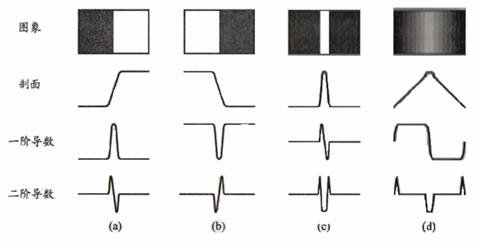2013-12-27 14:56:42 nedushy123 阅读数 2222
• ###### MATLAB图像处理

MATLAB图像处理课程

4588 人正在学习 去看看 李立宗

## 1.相关算子（Correlation Operator)

步骤：

1）滑动核，使其中心位于输入图像g的（i，j）像素上

2）利用上式求和，得到输出图像的（i，j）像素值

3）充分上面操纵，直到求出输出图像的所有像素值

例：

A =[17  24      15            h=[8     6
23      14  16                         7
13  20  22                         2]
10  12  19  21
11  18  25     9]

Matlab 函数：imfilter(A,h)

## 2.卷积算子（Convolution)

步骤：

1）将核围绕中心旋转180度

2）滑动核，使其中心位于输入图像g的（i，j）像素上

3）利用上式求和，得到输出图像的（i，j）像素值

4）充分上面操纵，直到求出输出图像的所有像素值

Matlab 函数：Matlab 函数：imfilter(A,h,'conv')%imfilter默认是相关算子，因此当进行卷积计算时需要传入参数'conv'

## 3.边缘效应

1）使用常数填充：imfilter默认用0填充，这会造成处理后的图像边缘是黑色的。

2）复制边缘像素：I3 = imfilter(I,h,'replicate');

## 4.常用滤波

fspecial函数可以生成几种定义好的滤波器的相关算子的核。

 1 2 3 4 5 `I = imread(``'moon.tif'``);` `h = fspecial(``'unsharp'``);` `I2 = imfilter(I,h);` `imshow(I), title(``'OriginalImage'``)` `figure, imshow(I2), title(``'FilteredImage'``)`

# 图像处理－线性滤波－2 图像微分（1、2阶导数和拉普拉斯算子）

## 1 一阶导数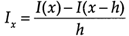，前向差分forwarddifferencing                  （1.2）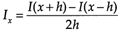，中心差分centraldifferencing                     （1.3）

1）前向差分的Matlab实现

 1 2 3 4 5 6 7 8 9 10 11 12 13 14 15 16 17 18 19 20 21 22 23 24 25 26 27 `function dimg = mipforwarddiff(img,direction)` `%MIPFORWARDDIFF     Finitedifferencecalculations ` `%` `%   DIMG= MIPFORWARDDIFF(IMG,DIRECTION)` `%` `%  Calculates theforward-difference` `for` ```agiven direction``` `%  IMG       :input image` `%  DIRECTION:` `'dx'` `or` `'dy'` `%  DIMG      :resultant image` `%` `%   Seealso MIPCENTRALDIFF MIPBACKWARDDIFFMIPSECONDDERIV` `%   MIPSECONDPARTIALDERIV` ` `  `%   OmerDemirkaya, Musa Asyali, Prasana Shaoo, ...9/1/06` `%   MedicalImage Processing Toolbox` ` `  `imgPad = padarray(img,[1 1],``'symmetric'``,``'both'``);%将原图像的边界扩展` `[row,col] = size(imgPad);` `dimg = zeros(row,col);` `switch` `(direction)   ` `case` `'dx'``,` `   ``dimg(:,1:col-1)=imgPad(:,2:col)-imgPad(:,1:col-1);%x方向差分计算，` `case` `'dy'``,` `   ``dimg(1:row-1,:)=imgPad(2:row,:)-imgPad(1:row-1,:); ` `otherwise, disp(````'Directionis unknown'````);` `end;` `dimg = dimg(2:end-1,2:end-1);`

2）中心差分的Matlab实现

 1 2 3 4 5 6 7 8 9 10 11 12 13 14 15 16 17 18 19 20 21 22 23 24 25 26 27 28 `function dimg = mipcentraldiff(img,direction)` `%MIPCENTRALDIFF     Finitedifferencecalculations ` `%` `%   DIMG= MIPCENTRALDIFF(IMG,DIRECTION)` `%` `%  Calculates thecentral-difference` `for` ```agiven direction``` `%  IMG       :input image` `%  DIRECTION:` `'dx'` `or` `'dy'` `%  DIMG      :resultant image` `%` `%   Seealso MIPFORWARDDIFF MIPBACKWARDDIFFMIPSECONDDERIV` `%   MIPSECONDPARTIALDERIV` ` `  `%   OmerDemirkaya, Musa Asyali, Prasana Shaoo, ...9/1/06` `%   MedicalImage Processing Toolbox` ` `  `img = padarray(img,[1 1],``'symmetric'``,``'both'``);` `[row,col] = size(img);` `dimg = zeros(row,col);` `switch` `(direction)` `    ``case` `'dx'``,` `        ````dimg(:,2:col-1)= (img(:,3:col)-img(:,1:col-2))/2;``` `    ``case` `'dy'``,` `        ````dimg(2:row-1,:)= (img(3:row,:)-img(1:row-2,:))/2;``` `    ``otherwise,` `        ``disp(````'Directionis unknown'````);` `end` `dimg = dimg(2:end-1,2:end-1);`
 1 ` `

 1 2 `I = imread(``'coins.png'````);figure; imshow(I);``` `Id = mipforwarddiff(I,``'dx'````);figure, imshow(Id);```

原图像                                                   x方向1阶导数

Matlab函数

2）quiver：以箭头形状绘制梯度。注意放大下面最右侧图可看到箭头，由于这里计算横竖两个方向的梯度，因此箭头方向都是水平或垂直的。

 1 2 3 4 5 `I =` `double``(imread(``'coins.png'``));` `[dx,dy]=gradient(I);` `magnitudeI=sqrt(dx.^2+dy.^2);` `figure;imagesc(magnitudeI);colormap(gray);%梯度幅值` `hold on;quiver(dx,dy);%叠加梯度方向`

梯度幅值                                   梯度幅值+梯度方向

## 3.1 普拉斯算子（laplacian operator）

2）因此，二阶差分为

### 3.1.2 应用

Matlab里有两个函数

1）del2

2）fspecial：图像处理中一般利用Matlab函数fspecial

h = fspecial('laplacian', alpha) returns a 3-by-3 filterapproximating the shape of the two-dimensional Laplacianoperator.
The parameter alpha controls the shape of the Laplacian and must bein the range 0.0 to 1.0. The default value for alpha is0.2.

### 3.1.3 资源

sift算法

Sift算法就是用不同尺度（标准差）的高斯函数对图像进行平滑，然后比较平滑后图像的差别，

sift可以同时处理亮度，平移，旋转，尺度的变化，利用特征点来提取特征描述符，最后在特征描述符之间寻找匹配

1构建尺度空间，检测极值点，获得尺度不变性

2特征点过滤并进行经确定位，剔除不稳定的特征点

3 在特征点处提取特征描述符，为特征点分配方向直

4声称特征描述子，利用特征描述符寻找匹配点

5计算变换参数

L(x,y,delta)=G(x,y,e)*i(x,y)

G(x,y,e) = [1/2*pi*e^2] * exp[ -(x^2 +y^2)/2e^2]

D(x,y,e)=(G(x,y,ke)-G(x,y,e))*i(x,y)

=L(x,y,ke)-L(x,y,e)

(为避免遍历每个像素点)

L(x,y,e)= G(x,y,e)*I(x,y)

图像金字塔的构建：图像金字塔共O组，每组有S层，下一组的图像由上一组图像降采样得到、

在尺度空间建立完毕后，为了能够找到稳定的关键点，采用高斯差分的方法来检测那些在局部位置的极值点，即采用俩个相邻的尺度中的图像相减，即公式定义为：
D(x,y,e)= ((G(x,y,ke) - G(x,y,e)) *I(x,y)
=L(x,y,ke) - L(x,y,e)
咱们再来具体阐述下构造D(x,y,e)的详细步骤：
1、首先采用不同尺度因子的高斯核对图像进行卷积以得到图像的不同尺度空间，将这一组图像作为金子塔图像的第一层。
2、接着对第一层图像中的2倍尺度图像（相对于该层第一幅图像的2倍尺度）以2倍像素距离进行下采样来得到金子塔图像的第二层中的第一幅图像，对该图像采用不同尺度因子的高斯核进行卷积，以获得金字塔图像中第二层的一组图像。
3、再以金字塔图像中第二层中的2倍尺度图像（相对于该层第一幅图像的2倍尺度）以2倍像素距离进行下采样来得到金字塔图像的第三层中的第一幅图像，对该图像采用不同尺度因子的高斯核进行卷积，以获得金字塔图像中第三层的一组图像。这样依次类推，从而获得了金字塔图像的每一层中的一组图像，
4、对上图得到的每一层相邻的高斯图像相减，就得到了高斯差分图像，如下述第一幅图所示。下述第二幅图中的右列显示了将每组中相邻图像相减所生成的高斯差分图像的结果，限于篇幅，图中只给出了第一层和第二层高斯差分图像的计算

——人（系统！）挨板子（脉冲！）以后，会有什么表现（输出！）？
——费话，疼呗！
——我问的是：会有什么表现？
——看疼到啥程度。像这无赖的体格，每天挨一个板子啥事都不会有，连哼一下都不可能，你也看到他那得意洋洋的嘴脸了（输出0）；如果一次连揍他十个板子，他可能会皱皱眉头，咬咬牙，硬挺着不哼
（输出1）；揍到二十个板子，他会疼得脸部扭曲，象猪似地哼哼（输出3）；揍到三十个板子，他可能会象驴似地嚎叫，一把鼻涕一把泪地求你饶他一命（输出5）；揍到四十个板子，他会大小便失禁，勉

——呜呼呀！这曲线象一座高山，弄不懂弄不懂。为啥那个无赖连挨了三十天大板却不喊绕命呀？
——呵呵，你打一次的时间间隔（Δτ=24小时）太长了，所以那个无赖承受的痛苦程度一天一利索，没有叠加，始终是一个常数；如果缩短打板子的时间间隔（建议Δτ=0.5秒），那他的痛苦程度可就迅速叠加了；等到这无赖挨三十个大板（t=30）时，痛苦程度达到了他能喊叫的极限，会收到最好的惩戒效果，再多打就显示不出您的仁慈了。
——还是不太明白，时间间隔小，为什么痛苦程度会叠加呢？
——这与人（线性时不变系统）对板子（脉冲、输入、激励）的响应有关。什么是响应？人挨一个板子后，疼痛的感觉会在一天（假设的，因人而异）内慢慢消失（衰减），而不可能突然消失。这样一来，只要打板子的时间间隔很小，每一个板子引起的疼痛都来不及完全衰减，都会对最终的痛苦程度有不同的贡献：
t个大板子造成的痛苦程度=Σ(第τ个大板子引起的痛苦*衰减系数)
[衰减系数是（t-τ）的函数，仔细品味]

——拿人的痛苦来说卷积的事，太残忍了。除了人以外，其他事物也符合这条规律吗？
——呵呵，县令大人毕竟仁慈。其实除人之外，很多事情也遵循此道。好好想一想，铁丝为什么弯曲一次不折，快速弯曲多次却会轻易折掉呢？
——恩，一时还弄不清，容本官慢慢想来——但有一点是明确地——来人啊，将撒尿的那个无赖抓来，狠打40大板！

1。你信号来的时候正赶上人家”系统”的响应时间段吗？
2。就算赶上系统响应时间段，响应有多少？

*********拉普拉斯*********

“拉 普拉斯变换” 就相当于上述例子中把数转换成”指数” 的过程；进行了拉普拉斯变换之后，复杂的微分方程(对应于上例中”复杂”的乘法)就变成了简单的代数方程，就象上例中”复杂”的乘法变成了简单的加减法。再把简单的代数方程的解反变换回去(就象把指数重新转换会一般的数一样)，就解决了原来那个复杂的微分方程。

1 。拉普拉斯变换之所以现在在电路中广泛应有，根本原因是电路中也广泛涉及了微分方程。
2。拉普拉斯变换与Z变换当然有紧密联系；其本质区别在于拉氏变换处理的是时间上连续的问题，Z变换处理的是时间上分立的问题。
Signals, Linear Systems, and Convolution

比如说你的老板命令你干活，你却到楼下打台球去了，后来被老板发现，他非常气愤，扇了你一巴掌（注意，这就是输入信号，脉冲），于是你的脸上会渐渐地（贱贱地）鼓起来一个包，你的脸就是一个系统，而鼓起来的包就是你的脸对巴掌的响应。
好，这样就和信号系统建立起来意义对应的联系。下面还需要一些假设来保证论证的严谨：假定你的脸是线性时不变系统，也就是说，无论什么时候老板打你一巴掌，打在你脸的同一位置（这似乎要求你的脸足够光滑，如果你说你长了很多青春痘，甚至整个脸皮处处连续处处不可导，那难度太大了，我就无话可说了），你的脸上总是会在相同的时间间隔内鼓起来一个相同高度的包来，并且假定以鼓起来的包的大小作为系统输出。好了，那么，下面可以进入核心内容——卷积了！
如果你每天都到楼下去打台球，那么老板每天都要扇你一巴掌，不过当老板打你一巴掌后，你5分钟就消肿了，所以时间长了，你甚至就适应这种生活了……如果有一天，老板忍无可忍，以0.5秒的间隔开始不间断的扇你的过程，这样问题就来了：第一次扇你鼓起来的包还没消肿，第二个巴掌就来了，你脸上的包就可能鼓起来两倍高，老板不断扇你，脉冲不断作用在你脸上，效果不断叠加了，这样这些效果就可以求和了，结果就是你脸上的包的高度岁时间变化的一个函数了（注意理解）！
如果老板再狠一点，频率越来越高，以至于你都辨别不清时间间隔了，那么，求和就变成积分了。可以这样理解，在这个过程中的某一固定的时刻，你的脸上的包的鼓起程度和什么有关呢？和之前每次打你都有关！但是各次的贡献是不一样的，越早打的巴掌，贡献越小，这就是说，某一时刻的输出是之前很多次输入乘以各自的衰减系数之后的叠加而形成某一点的输出，然后再把不同时刻的输出点放在一起，形成一个函数，这就是卷积。卷积之后的函数就是你脸上的包的大小随时间变化的函数。本来你的包几分钟就可以消肿，可是如果连续打，几个小时也消不了肿了，这难道不是一种平滑过程么？反映到公式上，f(a)就是第a个巴掌，g(x-a)就是第a个巴掌在x时刻的作用程度，乘起来再叠加就ok了，这就是卷积！
最后提醒各位，请勿亲身尝试……

3.1 一维卷积的定义

定义。这里参量x和积分变量α皆为实数；函数f和h可实可复。

1.1 一维傅立叶变换的定义与傅立叶积分定理

卷积公式是用来求随机变量和的密度函数(pdf)的计算公式。
定义式：
z(t)=x(t)*y(t)= ∫x(m)y(t-m)dm.
已知x,y的pdf,x(t),y(t).现在要求z=x+y的pdf. 我们作变量替显，令
z=x+y,m=x. 雅可比行列式=1.那么,t，m联合密度就是f(z,m)=x(m)y(z-m)*1.这样，就可以很容易求Z的在（z,m)中边缘分布
即fZ(z)=∫x(m)y(z-m)dm..... 由于这个公式和x(t),y(t)存在一一对应的关系。为了方便，所以记∫x(m)y(z-m)dm=x(t)*y(t)

for(i=0; i<N; i++)
{
for(j=0; j<N; j++)
{
g[i*N+j]=exp(-((i-(N-1)/2)^2+(j-(N-1)/2)^2))/(2*delta^2));
sum += g[i*N+j];
}
}

N是滤波器的大小，delta自选